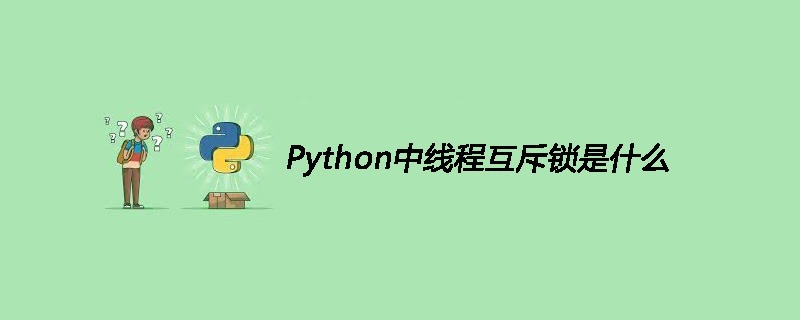## Python中线程互斥锁是什么

318次阅读1.线程互斥锁介绍```#创建锁
#锁定
mutex.acquire([blocking])
#释放
mutex.release()```

```from threading import Thread, Lock
import time
g_num = 0
def test1():
global g_num
for i in range(1000000):
#True表示堵塞 即如果这个锁在上锁之前已经被上锁了，那么这个线程会在这里一直等待到解锁为止
#False表示非堵塞，即不管本次调用能够成功上锁，都不会卡在这,而是继续执行下面的代码
mutexFlag = mutex.acquire(True)
if mutexFlag:#锁住
g_num += 1
mutex.release()#解锁
print("---test1---g_num=%d"%g_num)
def test2():
global g_num
for i in range(1000000):
mutexFlag = mutex.acquire(True) #True表示堵塞
if mutexFlag:#锁住
g_num += 1
mutex.release()#解锁
print("---test2---g_num=%d"%g_num)
#创建一个互斥锁
#这个所默认是未上锁的状态
mutex = Lock()
p1.start()
p2.start()
time.sleep(5)
print("---g_num=%d---"%g_num)```

```---test1---g_num=1942922
---test2---g_num=2000000
---g_num=2000000---```

2.上锁解锁过程

3.死锁

```#coding=utf-8
import time
def run(self):
if mutexA.acquire():
print(self.name+'----do1---up----')
time.sleep(1)
if mutexB.acquire():
print(self.name+'----do1---down----')
mutexB.release()
mutexA.release()
def run(self):
if mutexB.acquire():
print(self.name+'----do2---up----')
time.sleep(1)
if mutexA.acquire():
print(self.name+'----do2---down----')
mutexA.release()
mutexB.release()
if __name__ == '__main__':
t1.start()
t2.start()```

```Thread-1----do1---up----4.避免死锁